## Selina Concise Mathematics class 7 ICSE Solutions – Exponents (Including Laws of Exponents)

APlusTopper.com provides step-by-step solutions for Selina Concise ICSE Solutions for Class 7 Mathematics. You can download the Selina Concise Mathematics ICSE Solutions for Class 7 with a Free PDF download option. Selina Publishers Concise Mathematics for Class 7 ICSE Solutions all questions are solved and explained by expert mathematic teachers as per ICSE board guidelines.

EXERCISE 5 (A)

Question 1.
Find the value of:
(i) 6²
(ii) 73
(iii) 44
(iv) 55
(v) 83
(vi) 75

Solution:
(i) 62 = 6 x 6 = 36
(ii) 73 = 7 x 7 x 7 = 343
(iii) 44 = 4 x 4 x 4 x 4 = 256
(iv) 55= 5 x 5 x 5 x 5 x 5 = 3125
(v) 83 = 8 x 8 x 8 = 512
(vi) 7= 7 x 7 x 7 x 7 x 7 =16807

Question 2.
Evaluate:
(i) 23 x 42
(ii) 23 x 52
(iii) 33 x 52
(iv) 22 x 33
(v) 32 x 52
(vi) 53 x 24
(vii) 3x 42
(ix) (5 x 4)2

Solution:
(i) 23 x 42
= 2 x 2 x 2 x 4 x 4
= 8 x 16
= 128
(ii) 23 x 52
= 2 x 2 x 2 x 5 x 5
= 8 x 25
= 200
(iii) 33 x 52
=3 x 3 x 3 x 5 x 5
= 27 x 25
= 675
(iv) 22 x 33
= 2 x 2 x 3 x 3 x 3
= 4 x 27
= 108
(v) 32 x 53
=3 x3 x 5 x 5 x 5
= 9 x 125
= 1125
(vi) 53 x 24
= 5 x 5 x 5 x 2 x 2 x 2 x 2
= 125 x 16
= 2000
(vii) 32 x 42
=3 x 3 x 4 x 4
= 9 x 16
=144
(viii) (4 x 3)3
=4 x 4 x 4 x 3 x 3 x 3
= 64 x 27
= 1728
(ix) (5 x 4)2
=5 x 5 x 4 x 4
= 25 x 16
= 400

Question 3.
Evaluate: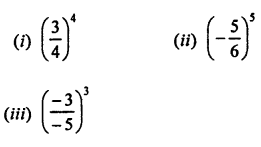Solution: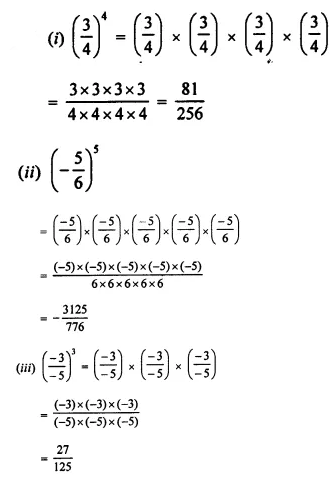Question 4.
Evaluate :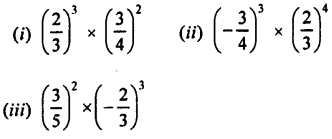Solution:Question 5.
Which is greater :
(i) 23 or 32
(ii) 25 or 52

(iii) 43 or 34
(iv) 54 or 45

Solution:
(i) 23 or 33
Since, 23 = 2 x 2 x 2 = 8
and, 32 = 3 x 3 = 9
∵9 is greater than 8 ⇒ 32 > 23
(ii) 25 or 52
Since, 25 = 2 x 2 x 2 x 2 x 2 = 32
and, 52 = 5 x 5 = 25
∵32 is greater than 25 ⇒ 235 > 532
(iii) 43 or 34
Since, 43 = 4 x 4 x 4 = 64
and, 34 = 3 x 3 x 3 x 3 = 81
∵ 81 is greater than 64 ⇒ 34 > 43
(iv) 54 or 45
Since, 54 = 5 x 5 x 5 x 5 = 625
and, 4= 4 x 4 x 4 x 4 x 4= 1024
∵ 1024 is greater than 625 ⇒ 45 > 54

Question 6.
Express each of the following in exponential form :
(i) 512
(ii) 1250
(iii) 1458
(iv) 3600
(v) 1350
(vi) 1176

Solution:
(i) 512(ii) 1250(iii) 1458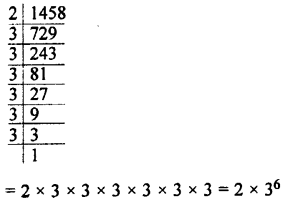(iv) 3600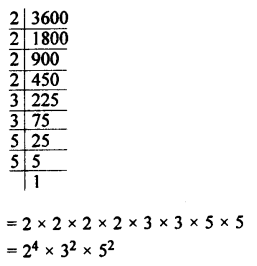(v) 1350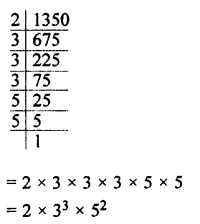(vi) 1176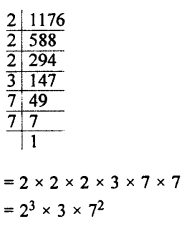Question 7.
If a = 2 and b = 3, find the value of:
(i) (a + b)2
(ii) (b – a)3
(iii) (a x b)a (iv) (a x b)b

Solution:
(i) (a + b)2
= (2 + 3)2 = (5)2 = 5 x 5 = 25

(ii) (b – a)2
= (3 – 2)2= (1)3
= 1 x 1 x 1 = 1

(iii) (a x b)a
= (2 x 3)2 – (6)2
= 6 x 6 = 36

(iv) (a x b)b
= (2 x 3)3 = (6)3 = 6 x 6 x 6 = 216

Question 8.
Express:
(i) 1024 as a power of 2.
(ii) 343 as a power of 7.
(iii) 729 as a power of 3.
Solution:
(i) 1024 as a power of 2.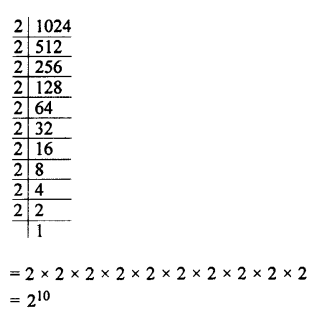(ii) 343 as a power of 7.(iii) 729 as a power of 3.Question 9.
If 27 x 32 = 3x x 2y; find the values of x and y.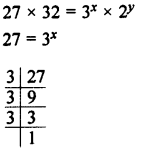Solution: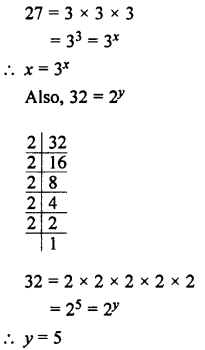Question 10.
If 64 x 625 = 2a x 5b; find :
(i) the values of a and b.
(ii) 2b x 5a

Solution:
(i) the values of a and b.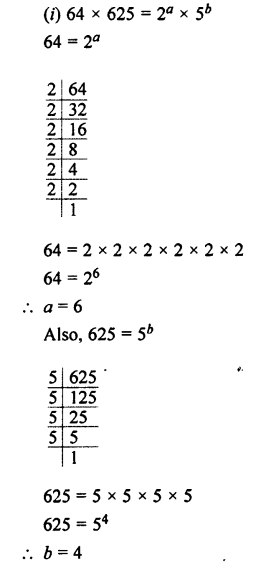(ii) 2b x 5a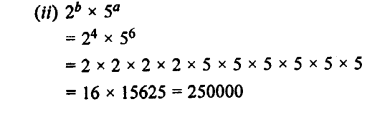EXERCISE 5 (B)

Question 1.
Fill in the blanks:
In 52 = 25, base = ……… and index = ……….
If index = 3x and base = 2y, the number = ………

Solution:
(i) In 52 = 25, base = 5 and index = 2
(ii) If index = 3x and base = 2y, the number = 2y3x

Question 2.
Evaluate:
(i) 28 ÷ 23
(ii) 2 28
(iii) (26)0
(iv) (3o)6
(v) 83 x 8-5 x 84
(vi) 5 x 53 + 55
(vii) 54 ÷ 53 x 55
(viii) 44 ÷ 43 x 40
(ix) (35 x 47 x 58)0

Solution:Question 3.
Simplify, giving Solutions with positive index: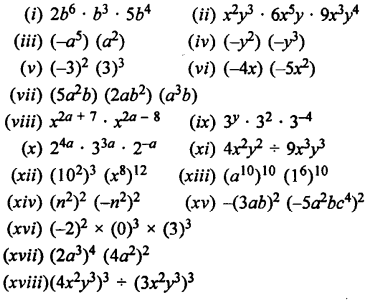Solution: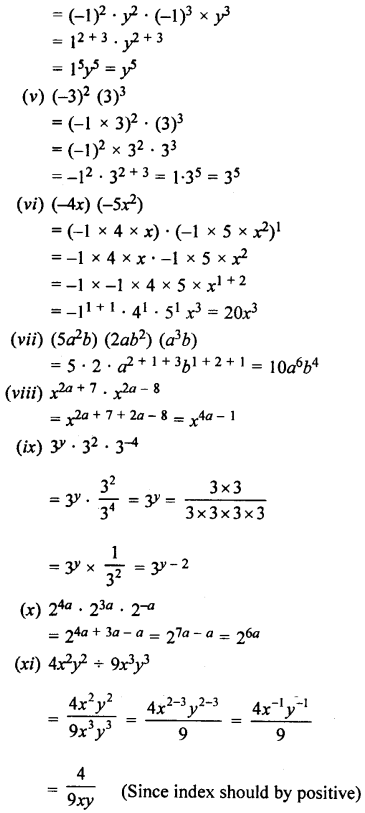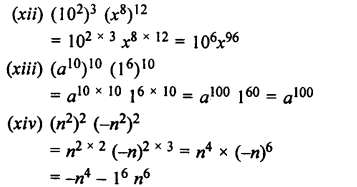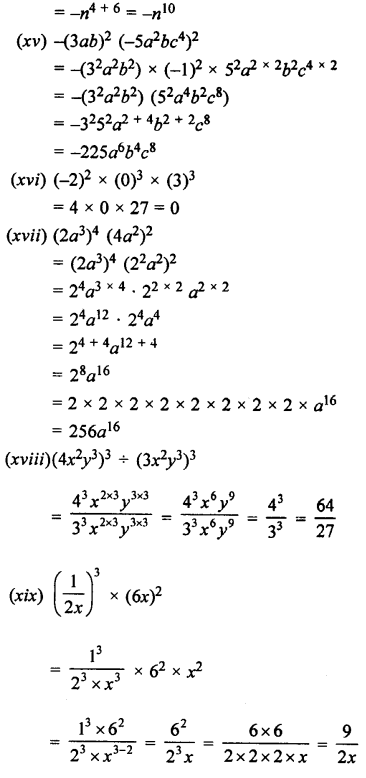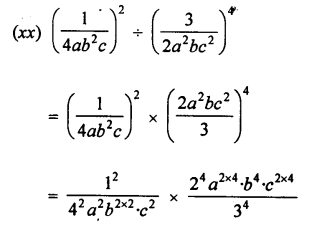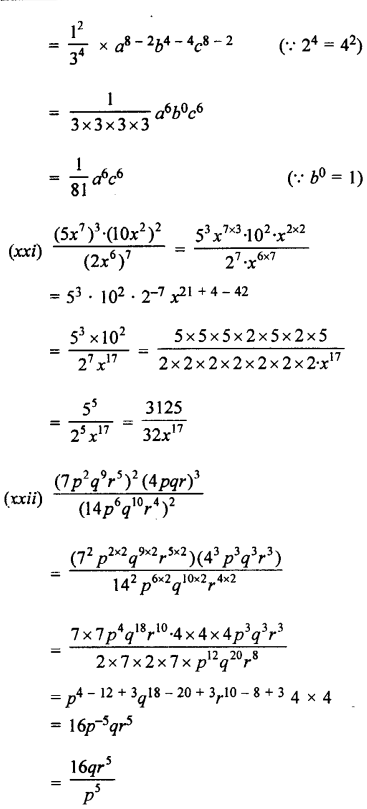Question 4.
Simplify and express the Solution in the positive exponent form :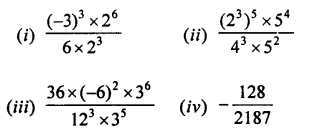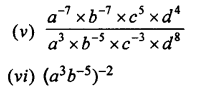Solution:Question 5.
Evaluate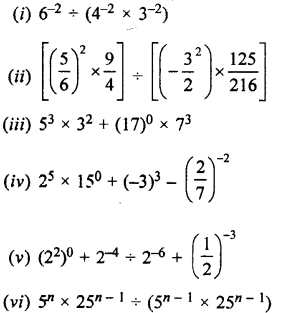Solution: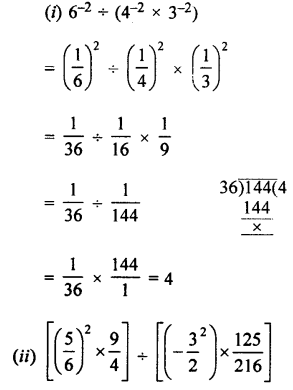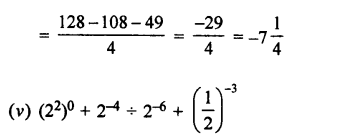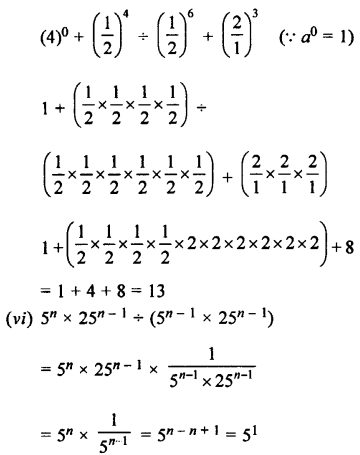Question 6.
If m2 = -2 and n = 2; find the values of:
(i) m + r2 – 2mn
(ii) mn + nm
(iii) 6m-3 + 4n2
(iv) 2n3 – 3m

Solution: